### D.18 Eigen­func­tions of com­mut­ing op­er­a­tors

Any two op­er­a­tors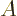and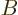that com­mute,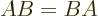, have a com­mon set of eigen­func­tions, pro­vided only that each has a com­plete set of eigen­func­tions. (In other words, the op­er­a­tors do not nec­es­sar­ily have to be Her­mit­ian. Uni­tary, anti-Her­mit­ian, etcetera, op­er­a­tors all qual­ify.)

First note the fol­low­ing:

if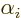is an eigen­func­tion ofwith eigen­value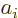, then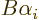is ei­ther also an eigen­func­tion ofwith eigen­valueor is zero.
To see that, note that sinceandcom­mute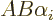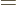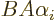which is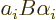. Com­par­ing start and end, the com­bi­na­tionmust be an eigen­func­tion ofwith eigen­valueif it is not zero. (Eigen­func­tions may not be zero.)

Now as­sume that there is just a sin­gle in­de­pen­dent eigen­func­tionfor each dis­tinct eigen­valueof. Then ifis nonzero, it can only be a mul­ti­ple of that sin­gle eigen­func­tion. By de­f­i­n­i­tion, that makesan eigen­func­tion oftoo, with as eigen­value the mul­ti­ple. On the other hand, ifis zero, thenis still an eigen­func­tion of, now with eigen­value zero. So un­der the stated as­sump­tion,andhave the ex­act same eigen­func­tions, prov­ing the as­ser­tion of this de­riva­tion.

How­ever, fre­quently there is de­gen­er­acy, i.e. there is more than one eigen­func­tion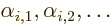for a sin­gle eigen­value. Then the fact that, say,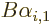is an eigen­func­tion ofwith eigen­valueno longer means thatis a mul­ti­ple of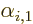; it only means thatis some com­bi­na­tion of all of. Which means thatis not in gen­eral an eigen­func­tion of.

To deal with that, it has to be as­sumed that the prob­lem has been nu­mer­i­cally ap­prox­i­mated by some fi­nite-di­men­sion­al one. Thenandwill be ma­tri­ces, and the num­ber of in­de­pen­dent eigen­func­tions (or rather, eigen­vec­tors now) ofandwill be fi­nite and equal. That al­lows the prob­lem to be ad­dressed one eigen­func­tion at a time.

As­sume now that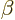is an eigen­func­tion of, with eigen­value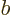, that is not yet an eigen­func­tion oftoo. By com­plete­ness, it can still be writ­ten as a com­bi­na­tion of the eigen­func­tions of, and more par­tic­u­larly as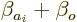where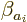is a com­bi­na­tion of the eigen­func­tions ofwith eigen­valueand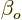a com­bi­na­tion of the eigen­func­tions ofwith other eigen­val­ues. There must be such eigen­func­tions withnonzero, be­cause with­out us­ing theyou can­not cre­ate an equal num­ber of in­de­pen­dent eigen­func­tions ofas of. By de­f­i­n­i­tion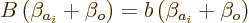but that must mean that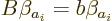since if it is not,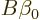can­not make up the dif­fer­ence; as seen ear­lier,only con­sists of eigen­func­tions ofthat do not have eigen­value. Ac­cord­ing to the above equa­tion,, which is al­ready an eigen­func­tion ofwith eigen­value, is also an eigen­func­tion ofwith eigen­value. So re­place one of the,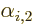,...with. (If you writein terms of the,,..., then the func­tion you re­place may not ap­pear with a zero co­ef­fi­cient.) Sim­i­larly re­place an eigen­func­tion ofwith eigen­valuewith. Thenandhave one more com­mon eigen­func­tion. Keep go­ing in this way and even­tu­ally all eigen­func­tions ofare also eigen­func­tions ofand vice versa.

Sim­i­lar ar­gu­ments can be used re­cur­sively to show that more gen­er­ally, a set of op­er­a­tors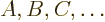that all com­mute have a sin­gle com­mon set of eigen­func­tions. The trick is to de­fine an ar­ti­fi­cial new op­er­a­tor, call it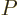, that has the com­mon eigen­func­tions ofand, but whose eigen­val­ues are dis­tinct for any two eigen­func­tions un­less these eigen­func­tions have the same eigen­val­ues for bothand. Then the eigen­func­tions of, even if you mess with them, re­main eigen­func­tions ofand. So go find com­mon eigen­func­tions forand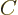.

The above de­riva­tion as­sumed that the prob­lem was fi­nite-​di­men­sion­al, or dis­cretized some way into a fi­nite-di­men­sion­al one like you do in nu­mer­i­cal so­lu­tions. The lat­ter is open to some sus­pi­cion, be­cause even the most ac­cu­rate nu­mer­i­cal ap­prox­i­ma­tion is never truly ex­act. Un­for­tu­nately, in the in­fi­nite-​di­men­sion­al case the de­riva­tion gets much trick­ier. How­ever, as the hy­dro­gen atom and har­monic os­cil­la­tor eigen­func­tion ex­am­ples in­di­cate, typ­i­cal in­fi­nite sys­tems in na­ture do obey the the­o­rem any­way.